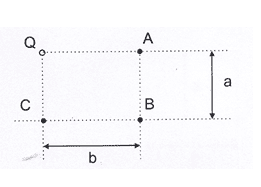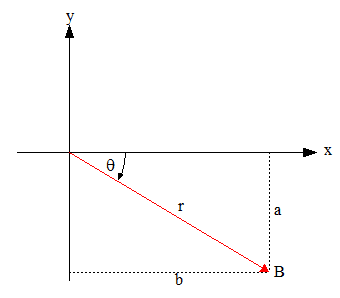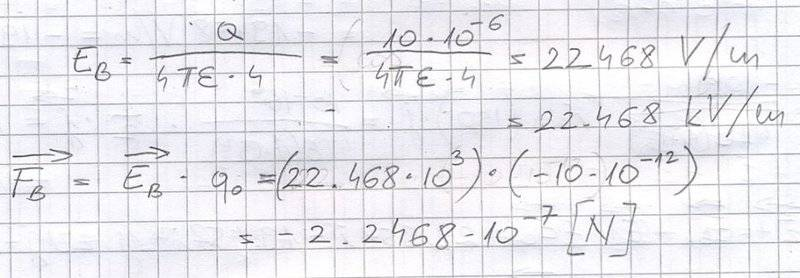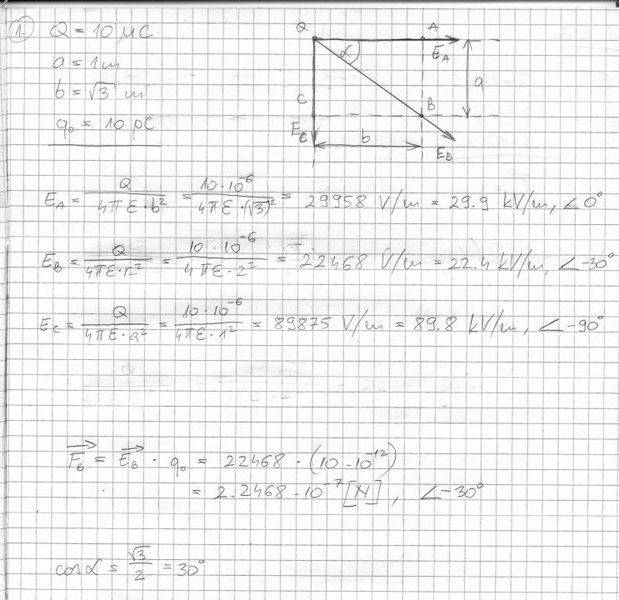# [College Physics] Electric field and point charge

## Homework Statement

Around a point charge there are three points: A, B and C. Determine the electric field vector for those points. Also, determine the force vector for q0 if it was in point B.

Q = 10 µC, a = 1 m, b = sqrt(3) m, q0 = 10 pC2. The attempt at a solution
Here is the attempt at a solution, and here are the correct answers.

EDIT: I can't find what I'm doing wrong but I'm not sure how do I get Eb from Ebx and Eby.

Last edited:

gneill
Mentor
Because the problem requires knowledge of the physical layout of points and charges you need to provide a visible diagram of the problem layout or a detailed description in the problem statement that makes the layout clear.

If you use the UPLOAD feature to upload an image as an attachment you can insert the image inline in your post. Often you can also simply paste an image captured via a screen clipping tool (such as "Snipping Tool" on Windows platforms).

Because the problem requires knowledge of the physical layout of points and charges you need to provide a visible diagram of the problem layout or a detailed description in the problem statement that makes the layout clear.

If you use the UPLOAD feature to upload an image as an attachment you can insert the image inline in your post. Often you can also simply paste an image captured via a screen clipping tool (such as "Snipping Tool" on Windows platforms).
Yeah, sorry I'm first time here, I edited it.

gneill
Mentor
EDIT: I can't find what I'm doing wrong but I'm not sure how do I get Eb from Ebx and Eby.

That's kind of the long way to get to Eb. You are given enough information to find the force magnitude and angle separately.You can determine the length of the vector r easily enough from the given components. From there you can find the magnitude of the electric field. You can also determine the angle θ from the components.

It looks like I'm forgeting something. Where do I use the angle θ?gneill
Mentor
It looks like I'm forgeting something. Where do I use the angle θ?
View attachment 108763
The field and force are both vectors. Thus they have magnitude and direction. A vector can be expressed in Cartesian component form (i, j, k components), or in polar form (magnitude and angle). Finding the polar form for the field and force at point b is straightforward.

In post #4 the red vector represents the position vector r for point b. You can find its magnitude and angle by Pythagoras and a bit of trig using the side lengths of the triangles formed.

The electric field magnitude depends on the distance magnitude (Coulomb's Law formula). The angle will be the same as for the position vector. Similarly the magnitude of the force on some charge q placed at location b can be found using the magnitude of the electric field there. Again the angle associated with the force vector will be given by that of the electric field angle (and position vector angle). The only caveat to that being if the charge is negative then the direction of the resulting force will be flipped in direction 180°.gneill
Mentor
That looks good.